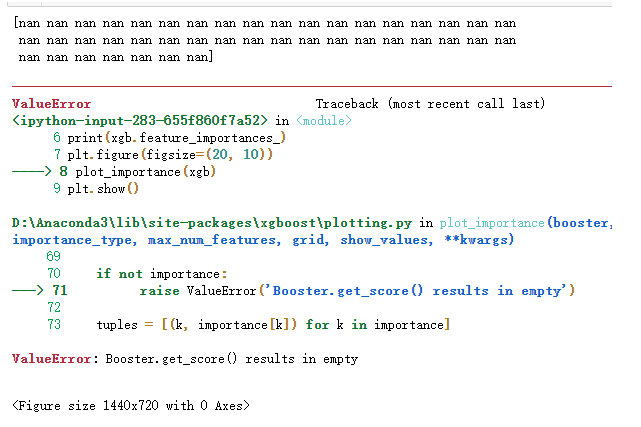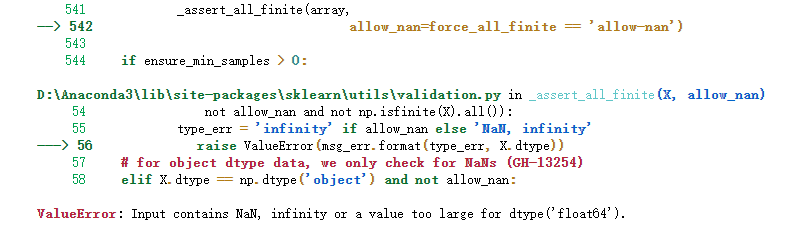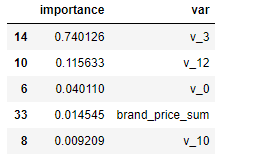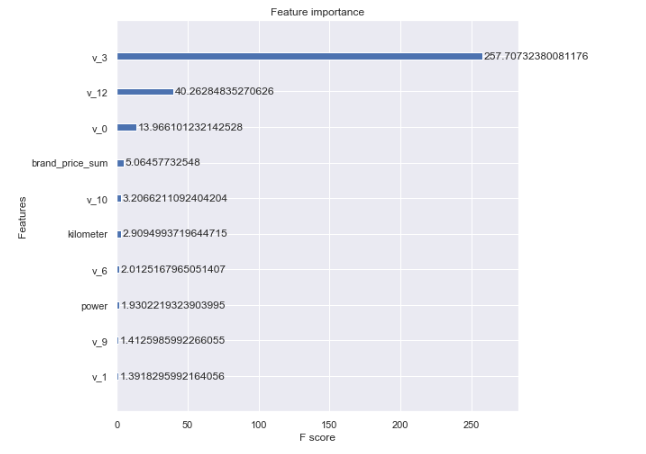• # XX为df格式 train特征表 feature_names = XX.columns.tolist() feature_names = np.array(feature_names) feature_importances = clf.feature_importances_ indices = np.argsort(feature_importances)[::-1] x = ...
# XX为df格式 train特征表
feature_names = XX.columns.tolist()
feature_names = np.array(feature_names)
feature_importances = clf.feature_importances_
indices = np.argsort(feature_importances)[::-1]
x = feature_importances[show_indices]
y=feature_names[indices]


展开全文• 这里尝试通过查看特征重要性来筛选特征： from xgboost import XGBRegressor from xgboost import plot_importance xgb = XGBRegressor() xgb.fit(X, Y) print(xgb.feature_importances_) plt.figure(figsize=(20, ...
• 1.输出XGBoost特征重要性 from matplotlib import pyplot pyplot.bar(range(len(model_XGB.feature_importances_)), model_XGB.feature_importances_) pyplot.show() XGBoost 特征重要性绘图 也可以使用...
1.输出XGBoost特征的重要性
from matplotlib import pyplot
pyplot.bar(range(len(model_XGB.feature_importances_)), model_XGB.feature_importances_)
pyplot.show()
XGBoost 特征重要性绘图
也可以使用XGBoost内置的特征重要性绘图函数
# plot feature importance using built-in function
from xgboost import plot_importance
plot_importance(model_XGB)
pyplot.show()
XGBoost 内置的特征重要性绘图
2.根据特征重要性筛选特征
from numpy import sort
from sklearn.feature_selection import SelectFromModel

# Fit model using each importance as a threshold
thresholds = sort(model_XGB.feature_importances_)
for thresh in thresholds:
# select features using threshold
selection = SelectFromModel(model_XGB, threshold=thresh, prefit=True)
select_X_train = selection.transform(X_train)
# train model
selection_model = XGBClassifier()
selection_model.fit(select_X_train, y_train)
# eval model
select_X_test = selection.transform(X_test)
y_pred = selection_model.predict(select_X_test)
predictions = [round(value) for value in y_pred]
accuracy = accuracy_score(y_test, predictions)
print("Thresh=%.3f, n=%d, Accuracy: %.2f%%" % (thresh, select_X_train.shape,
accuracy*100.0))
XGBoost 筛选特征
参考：https://blog.csdn.net/u011630575/article/details/79423162
展开全文XGBoost
• 1 问题描述 我想用XGBoost来建立一个模型，通过特征...这里尝试通过查看特征重要性来筛选特征： from xgboost import XGBRegressor from xgboost import plot_importance xgb = XGBRegressor() xgb.fit(X, Y) prin...
1 问题描述
我想用XGBoost来建立一个模型，通过特征构造之后我需要做一个特征选择来减少特征数量、降维，使模型泛化能力更强，减少过拟合：
这里尝试通过查看特征重要性来筛选特征：
from xgboost import XGBRegressor
from xgboost import plot_importance

xgb = XGBRegressor()
xgb.fit(X, Y)
print(xgb.feature_importances_)

plt.figure(figsize=(20, 10))
plot_importance(xgb)
plt.show()

输出如下：这里输出的特征重要性全部为 ‘nan’，画图也抛出了一个错误：ValueError:Booster.get_score() results in empty
2 问题原因
查了一下plot_importance(）的源码，截取了相关部分如下：
    if len(importance) == 0:
raise ValueError('Booster.get_score() results in empty')

说明这里画图画不出来也是因为输出的特征重要性全部为’nan’。 通过一下午的debug我发现问题出现在响应变量Y当中：
Y.isnull().sum()

输出为：
5000

Y出现了5000个空值，我才想起在做特征工程的时候为了方便，将训练集和测试集连接到一起了，而测试集中的5000个样本的响应变量都是’nan’。 事实上，监督机器学习算法在训练的过程中，如果遇到响应变量为’nan’，很多算法都会出错，下面以简单的线性回归为例：
import numpy as np
import pandas as pd
import sklearn
from sklearn.linear_model import LinearRegression

y = np.array([0, 1, 2, 3, np.nan, 5, 6, 7, 8, 9])
x = np.random.rand(len(y),3)

LR = LinearRegression()
LR.fit(x,y)

会抛出错误：3 解决方案
这里我是因为将训练集和测试集合并到一起了造成响应变量中出现’nan’,所以直接分开就可以了。如果训练集的响应变量本身就含有‘nan’，如果这类样本非常少，可以考虑删掉，如果是分类问题，可以考虑将这类样本单独作为一个类。
from xgboost import XGBRegressor
from xgboost import plot_importance

xgb = XGBRegressor()
xgb.fit(X, Y)

im=pd.DataFrame({'importance':xgb.feature_importances_,'var':X.columns})
im=im.sort_values(by='importance',ascending=False)

输出如下：fig,ax = plt.subplots(figsize=(8,8))
plot_importance(xgb,max_num_features=10,ax=ax,importance_type='gain')
plt.show()展开全文机器学习 python 数据挖掘
• XGBoost输出特征重要性以及筛选特征 1，梯度提升算法是如何计算特征重要性的？ 使用梯度提升算法的好处是在提升树被创建后，可以相对直接地得到每个属性的重要性得分。一般来说，重要性分数，衡量了特征在模型中的...
XGBoost学习（一）：原理 XGBoost学习（二）：安装及介绍 XGBoost学习（三）：模型详解 XGBoost学习（四）：实战 XGBoost学习（五）：参数调优 XGBoost学习（六）：输出特征重要性以及筛选特征 完整代码及其数据
XGBoost输出特征重要性以及筛选特征
1，梯度提升算法是如何计算特征重要性的？
使用梯度提升算法的好处是在提升树被创建后，可以相对直接地得到每个属性的重要性得分。一般来说，重要性分数，衡量了特征在模型中的提升决策树构建中的价值。一个属性越多的被用来在模型中构建决策树，它的重要性就相对越高。
属性重要性是通过对数据集中的每个属性进行计算，并进行排序得到。在单个决策树中通过每个属性分裂点改进性能度量的量来计算属性重要性。由节点负责加权和记录次数，也就是说一个属性对分裂点改进性能度量越大（越靠近根节点），权值越大；被越多提升树所选择，属性越重要。性能度量可以是选择分裂节点的Gini纯度，也可以是其他度量函数。
最终将一个属性在所有提升树中的结果进行加权求和后然后平均，得到重要性得分。
2，绘制特征重要性
一个已训练的Xgboost模型能够自动计算特征重要性，这些重要性得分可以通过成员变量feature_importances_得到。可以通过如下命令打印：
print(model.feature_importances_)

我们可以直接在条形图上绘制这些分数，以便获得数据集中每个特征的相对重要性的直观显示，例如：
# plot
pyplot.bar(range(len(model.feature_importances_)), model.feature_importances_)
pyplot.show()

我们可以通过在the Pima Indians onset of diabetes 数据集上训练XGBoost模型来演示，并从计算的特征重要性中绘制条形图。
# plot feature importance manually
from xgboost import XGBClassifier
from matplotlib import pyplot
# split data into X and y
X = dataset.data
y = dataset.target
# fit model no training data
model = XGBClassifier()
model.fit(X, y)
# feature importance
print(model.feature_importances_)
# plot
pyplot.bar(range(len(model.feature_importances_)), model.feature_importances_)
pyplot.show()

运行这个实例，首先输出特征重要性分数： [0.17941953 0.11345647 0.41556728 0.29155672] 相对重要性条形图：这种绘制的缺点在于，只显示了特征重要性而没有排序，可以在绘制之前对特征重要性得分进行排序。 　　通过内建的绘制函数进行特征重要性得分排序后的绘制，这个函数就是plot_importance()，示例如下：
# plot feature importance manually
from xgboost import XGBClassifier
from matplotlib import pyplot
from xgboost import plot_importance

# split data into X and y
X = dataset.data
y = dataset.target
# fit model no training data
model = XGBClassifier()
model.fit(X, y)
# feature importance
print(model.feature_importances_)
# plot feature importance

plot_importance(model)
pyplot.show()

示例得到条形图：根据其在输入数组的索引，特征被自动命名为f0~f3，在问题描述中手动的将这些索引映射到名称，我们可以看到，f2具有最高的重要性，f1具有最低的重要性。
3，根据Xgboost特征重要性得分进行特征选择
特征重要性得分，可以用于在scikit-learn中进行特征选择。通过SelectFromModel类实现，该类采用模型并将数据集转换为具有选定特征的子集。这个类可以采取预先训练的模型，例如在整个数据集上训练的模型。然后，它可以阈值来决定选择哪些特征。当在SelectFromModel实例上调用transform()方法时，该阈值被用于在训练集和测试集上一致性选择相同特征。
在下面的示例中，我们首先在训练集上训练xgboost模型，然后在测试上评估。使用从训练数据集计算的特征重要性，然后，将模型封装在一个SelectFromModel实例中。我们使用这个来选择训练集上的特征，用所选择的特征子集训练模型，然后在相同的特征方案下对测试集进行评估。
# select features using threshold
selection = SelectFromModel(model, threshold=thresh, prefit=True)
select_X_train = selection.transform(X_train)
# train model
selection_model = XGBClassifier()
selection_model.fit(select_X_train, y_train)
# eval model
select_X_test = selection.transform(X_test)
y_pred = selection_model.predict(select_X_test)

我们可以通过测试多个阈值，来从特征重要性中选择特征。具体而言，每个输入变量的特征重要性，本质上允许我们通过重要性来测试每个特征子集。
完整代码如下：
# plot feature importance manually
import numpy as np
from xgboost import XGBClassifier
from matplotlib import pyplot
from xgboost import plot_importance
from sklearn.model_selection import train_test_split
from sklearn.metrics import accuracy_score
from sklearn.feature_selection import SelectFromModel

# split data into X and y
X = dataset.data
y = dataset.target

# split data into train and test sets
X_train,X_test,y_train,y_test = train_test_split(X,y,test_size=0.33,random_state=7)

# fit model no training data
model = XGBClassifier()
model.fit(X_train, y_train)
# feature importance
print(model.feature_importances_)

# make predictions for test data and evaluate
y_pred = model.predict(X_test)
predictions = [round(value) for value in y_pred]
accuracy = accuracy_score(y_test,predictions)
print("Accuracy:%.2f%%"%(accuracy*100.0))

#fit model using each importance as a threshold
thresholds = np.sort(model.feature_importances_)
for thresh in thresholds:
# select features using threshold
selection = SelectFromModel(model,threshold=thresh,prefit=True )
select_X_train = selection.transform(X_train)
# train model
selection_model = XGBClassifier()
selection_model.fit(select_X_train, y_train)
# eval model
select_X_test = selection.transform(X_test)
y_pred = selection_model.predict(select_X_test)
predictions = [round(value) for value in y_pred]
accuracy = accuracy_score(y_test,predictions)
print("Thresh=%.3f, n=%d, Accuracy: %.2f%%" % (thresh, select_X_train.shape, accuracy * 100.0))

运行示例，得到输出： [0.20993228 0.09029345 0.54176074 0.15801354] Accuracy:92.00% Thresh=0.090, n=4, Accuracy: 92.00% Thresh=0.158, n=3, Accuracy: 92.00% Thresh=0.210, n=2, Accuracy: 86.00% Thresh=0.542, n=1, Accuracy: 90.00% 我们可以看到，模型的性能通常随着所选择的特征的数量减少，在这一问题上，可以对测试集准确率和模型复杂度做一个权衡，例如选择三个特征，接受准确率为92%，这可能是对这样一个小数据集的清洗，但是对于更大的数据集和使用交叉验证作为模型评估方案可能是更有用的策略。
4，网格搜索
代码1：
from sklearn.model_selection import GridSearchCV
tuned_parameters= [{'n_estimators':[100,200,500],
'max_depth':[3,5,7], ##range(3,10,2)
'learning_rate':[0.5, 1.0],
'subsample':[0.75,0.8,0.85,0.9]
}]
tuned_parameters= [{'n_estimators':[100,200,500,1000]
}]
clf = GridSearchCV(XGBClassifier(silent=0,nthread=4,learning_rate= 0.5,min_child_weight=1, max_depth=3,gamma=0,subsample=1,colsample_bytree=1,reg_lambda=1,seed=1000), param_grid=tuned_parameters,scoring='roc_auc',n_jobs=4,iid=False,cv=5)
clf.fit(X_train, y_train)
##clf.grid_scores_, clf.best_params_, clf.best_score_
print(clf.best_params_)
y_true, y_pred = y_test, clf.predict(X_test)
print"Accuracy : %.4g" % metrics.accuracy_score(y_true, y_pred)
y_proba=clf.predict_proba(X_test)[:,1]
print "AUC Score (Train): %f" % metrics.roc_auc_score(y_true, y_proba)

代码2：
from sklearn.model_selection import GridSearchCV
parameters= [{'learning_rate':[0.01,0.1,0.3],'n_estimators':[1000,1200,1500,2000,2500]}]
clf = GridSearchCV(XGBClassifier(
max_depth=3,
min_child_weight=1,
gamma=0.5,
subsample=0.6,
colsample_bytree=0.6,
objective= 'binary:logistic', #逻辑回归损失函数
scale_pos_weight=1,
reg_alpha=0,
reg_lambda=1,
seed=27
),
param_grid=parameters,scoring='roc_auc')
clf.fit(X_train, y_train)
print(clf.best_params_)
y_pre= clf.predict(X_test)
y_pro= clf.predict_proba(X_test)[:,1]
print "AUC Score : %f" % metrics.roc_auc_score(y_test, y_pro)
print"Accuracy : %.4g" % metrics.accuracy_score(y_test, y_pre)

输出特征重要性：
import pandas as pd
import matplotlib.pylab as plt
feat_imp = pd.Series(clf.booster().get_fscore()).sort_values(ascending=False)
feat_imp.plot(kind='bar', title='Feature Importances')
plt.ylabel('Feature Importance Score')
plt.show()

补充：关于随机种子——random_state
random_state是一个随机种子，是在任意带有随机性的类或者函数里作为参数来控制随机模式。random_state取某一个值的时候，也就确定了一种规则。 random_state可以用于很多函数，比如训练集测试集的划分；构建决策树；构建随机森林
1，划分训练集和测试集的类train_test_split
随机数种子控制每次划分训练集和测试集的模式，其取值不变时划分得到的结果一模一样，其值改变时，划分得到的结果不同。若不设置此参数，则函数会自动选择一种随机模式，得到的结果也就不同。
2，构建决策树的函数
clf = tree.DecisionTreeClassifier(criterion="entropy",random_state=30,splitter="random")

其取值不变时，用相同的训练集建树得到的结果一模一样，对测试集的预测结果也是一样的 其取值改变时，得到的结果不同； 若不设置此参数（即设置为None），则函数会自动选择一种随机模式，每次得到的结果也就不同，可能稍微有所波动。
3，构建随机森林
clf = RandomForestClassifier(random_state=0)

其取值不变时，用相同的训练集建树得到的结果一模一样，对测试集的预测结果也是一样的 其取值改变时，得到的结果不同； 若不设置此参数（即设置为None），则函数会自动选择一种随机模式，每次得到的结果也就不同，可能稍微有所波动。
4，总结
在需要设置random_state的地方给其赋值，当多次运行此段代码得到完全一样的结果，别人运行代码也可以复现你的过程。若不设置此参数则会随机选择一个种子，执行结果也会因此不同。虽然可以对random_state进行调参，但是调参后再训练集上表现好的模型未必在陌生训练集上表现好，所以一般会随便选择一个random_state的值作为参数。 对于那些本质上是随机的过程，我们有必要控制随机的状态，这样才能重复的展现相同的结果。如果对随机状态不加控制，那么实验的结果就无法固定，而是随机的显示。 其实random_state 与 random seed作用是相同的，下面我们通过 random seed来学习一下 random_state：第一段代码和第二段代码完全相同，在1~100中取10个随机数，都没有设置 random seed，它每次取的结果就不太，它的随机数种子与当前系统的时间有关。 第三段代码和第四段代码设置了相同的 random seed(123)，他们取的随机数就完全相同，你多运行几次也是这样。 第五段代码设置了 random seed(456)，但是与之前设置的不同，于是运行取随机数的结果也不同。
展开全文机器学习 深度学习 人工智能
• 加载模型 import xgboost as xgb ...# 查看模型重要性 sorted([(i,v) for i,v in model.get_fscore().items()],key=lambda x:x) 结果如下： [………， ('f63', 396), ('f65', 397), ('f56', 405), ('f62',
• 在判断训练得到的模型是否合理时，一个很重要的步骤就是查看xgboost模型的特征重要性排序。如果观察得到模型的排名前几的特征都不符合我们正常的思维，那么模型很可能是不稳定或者有问题的。在训练得到模型文件及...
• 注意，下文的原始的...XGB知道吗，以XGB为例子说一下调参流程吧（个人的思路）：一般来说采用贝叶斯优化或者遗传算法等启发式的优化算法确定相对最佳参数（如果不熟悉的话用随机搜索也是可以的，或者网格搜索但是...
• 在使用GBDT、RF、Xgboost等树类模型建模时，往往可以通过 feature_importance 来返回特征重要性，各模型输出特征重要性的原理与方法 一 计算特征重要性方法 首先，目前计算特征重要性计算方法主要有两个方面： ...
• 1、gbdt、xgb 特征重要性源码解释：https://zhuanlan.zhihu.com/p/64759172 2、shap对xgboost特征重要性评估 https://zhuanlan.zhihu.com/p/64799119数据挖掘 机器学习
• 一行代码 plot_importance(model).set_yticklabels([feature_names]) 参考
• 很多时候我们输出特征重要性gain值和cover值不一致，会导致些许困惑（到底那个特征最为重要，那个特征重要性要靠前）。所以，我们考虑用shapely value 来衡量特征重要性，它即考虑了特征的cover，同时也考虑了...自然语言处理 深度学习 机器学习
• 最近在复习特征重要性时候，考虑到我们在使用GBDT、RF、Xgboost等树类模型建模时，往往可以通过 feature_importance 来返回特征重要性，下面收集整理了一下各模型输出特征重要性的原理与方法；树模型
• 用xgboost模型对特征重要性进行排序 在这篇文章中，你将会学习到： xgboost对预测模型特征重要性排序的原理（即为什么xgboost可以对预测模型特征重要性进行排序）。 如何绘制xgboost模型得到的特征重要性条形图。...
• 1.xgboost对特征重要性排序的原理 xgboost根据结构分数的增益情况计算出来选择哪个特征作为分割点,而某个特征重要性就是它在所有树中出现的次数之和。也就是说一个属性越多的被用来在模型中构建决策树，它的重要性...
• 运行此示例首先输出重要性分数。 [ 0.089701 0.17109634 0.08139535 0.04651163 0.10465116 0.2026578 0.1627907 0.14119601] 我们还得到了一个相对重要性的条形图。 这个图的一个缺点是特征是按输入索引而不是重要...python 机器学习 xgboost
• 我封装的多标签（multi-label）XGBoost模型是像下面这样写的： ...multi_xgb = MultiOutputRegressor(XGBRegressor(max_depth=10, learning_rate=0.1, n_estimators=500, silent=False)) multi_xgb.fit(trai
• GBDT和XGB基本上是机器学习面试里面的必考题。最近面试了五十场面试，基本三分之二的面试官都问了关于GBDT和XGB的问题。下面把里面常用的知识点、常见的面试题整理出来首先来说集成学习集成学习boosting 串行的方式...
• ## xgboost特征重要性

千次阅读 2019-02-16 21:56:58
silent=0 ,#设置成1则没有运行信息输出，最好是设置为0.是否在运行升级时打印消息。 learning_rate= 0.3, # 如同学习率 min_child_weight=1,  max_depth=6, # 构建树的深度，越大越容易过拟合 gamma=0, # 树的...
• 模型会自带一些输出重要属性的方法。 2.线性模型，模型一般可以直接输出参数，参数越大越重要。 3.利用互信息等类似的方法可以做，sklearn有，scipy里面也有。 l1正则，可以观察训练完毕后参数为零的特征，删除掉就...
• xgboost特征重要性指标: weight, gain, cover 文章转载自： https://blog.csdn.net/sujinhehehe/article/details/84201415 官方解释 Python中的xgboost可以通过get_fscore获取特征重要性，先看看官方对于这个方法的...机器学习 决策树 python...